# 数据结构与算法之美学习笔记

## 基础篇之排序(下)

Posted by Alee on October 16, 2019

## 基础篇之排序(下)

#### 归并排序的原理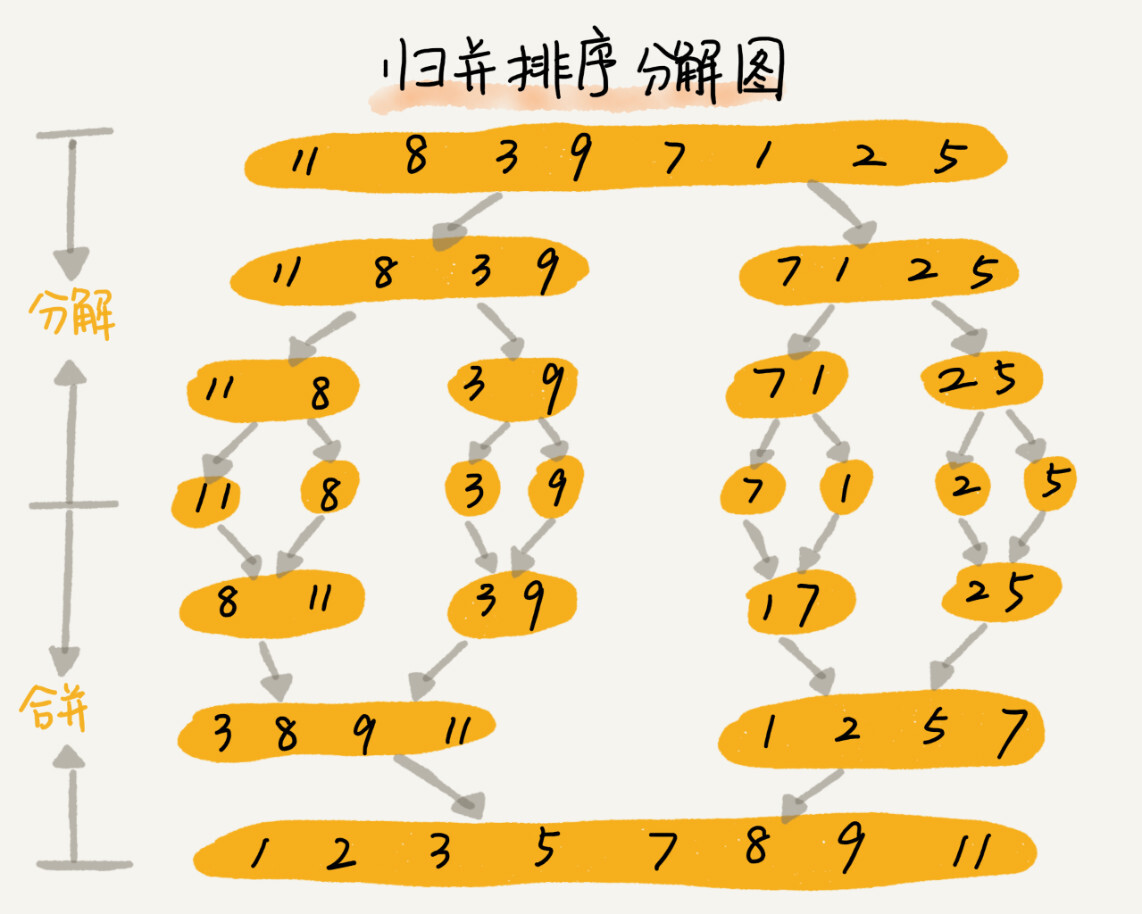``````//递推公式
merge_sort(p...r) = merge(merge_sort(p...q), merge_sort(q+1...r))
//终止条件
p >= r 不用再继续分解
``````

``````//归并排序算法， a 是数组，n 表示数组大小
public void mergeSort(int[] a, int n) {
mergeSortC(a, 0, n-1);
}
//递归调用函数
public void mergeSortC(int[] a, int p, int r) {
//递归终止条件
if (p >= r) return;

//取 p 到 r 之间的中间位置q
int q = (p + r) / 2;
//分治递归
mergeSortC(a, p, q);
mergeSortC(a, q+1, r);
//将a[p...q] 和 a[q+1...r] 合并为 a[p...r]
merge(a, p, r, q); //merge(a[p...r], a[p...q], a[q+1...r]);
}
``````

merge(a[p…r], a[p…q], a[q+1…r])这个函数的作用是将已经有序的a[p…q]和a[q+1…r]合并成一个有序数组，并且放入a[p…r]。那这个过程如何实现呢？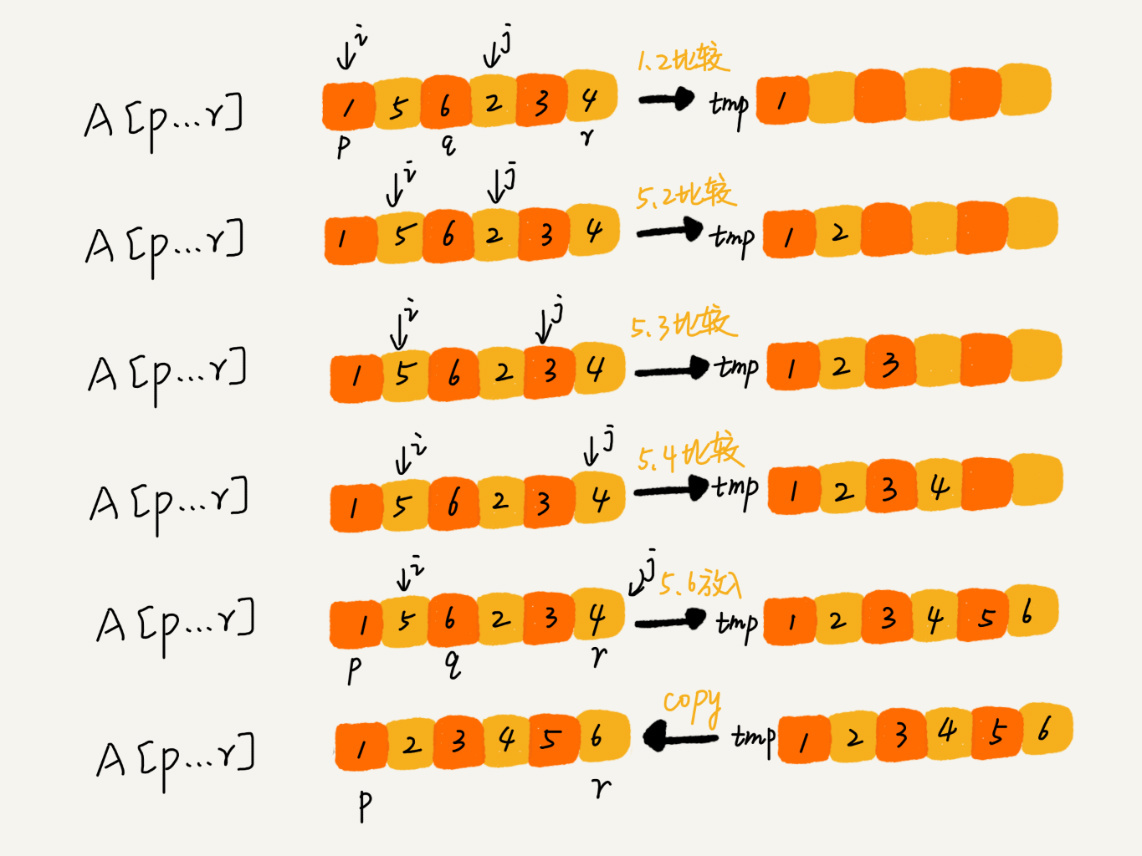``````public void merge(int[] a, int p, int r, int q) {
//初始化变量 i ，j，k
int i = p;
int j = q + 1;
int k = 0;
int[] tmp = new int[r-p+1]; //申请一个大小跟a[p...r]一样的临时数组
while(i <= q && j <= r) {
if (a[i] <= a[j]) {
tmp[k++] = a[i++];
} else {
tmp[k++] = a[j++];
}
}

//判断哪个子数组中有剩余的数据
int start = i, end = q;
if (j <= r) {
start = j;
end = r;
}
//将剩余的数据拷贝到临时数组tmp
while (start <= end) {
tmp[k++] = a[start++];
}
//将tmp中的数组拷贝回a[p...r]
for (i = 0; i <= r - p; i++) {
a[p+i] = tmp[i];
}
}
``````

#### 归并排序的性能分析

• 归并排序是稳定的排序算法吗？

归并排序稳不稳定关键要看merge()函数，也就是两个有序子数组合并成一个有序数组的那部分代码。

在合并的过程中，如果a[p…q]和a[q+1…r]之间有值相同的元素，那我们可以先把a[p…q]中的元素放入tmp数组。这样就保证了值相同的元素，在合并前后的先后顺序不变，所以，归并排序是一个稳定的排序算法。

• 归并排序的时间复杂度是多少？

归并排序涉及递归，递归的适用场景是，一个问题a可以分解为多个子问题b、c，那求解问题a就可以分解为求解问题b、c。问题b、c解决之后，我们再把b、c的结果合并成a的结果。

如果我们定义求解问题a的时间是T(a)，求解问题b、c的时间分别是T(b)和T(c)，那就可以得到这样的递推公式：

``````T(a) = T(b) + T(c) + K
``````

其中K等于将两个子问题b、c的结果合并成问题a的结果所消耗的时间。

由此得出一个重要的结论：不仅递归求解的问题可以写成递推公式，递归代码的时间复杂度也可以写成递推公式

我们假设对n个元素进行归并排序需要的时间是T(n)，那分解成两个子数组排序的时间都是T(n/2)。由于merge()函数合并两个有序子数组的时间复杂度是O(n)。所以套用前面的公式，归并排序的时间复杂度的计算公式为：

``````T(1) = C；   n=1 时，只需要常量级的执行时间，所以表示为 C。
T(n) = 2*T(n/2) + n； n>1
``````

通过这个公式，再进一步分解求解的计算过程。

``````T(n) = 2*T(n/2) + n
= 2*(2*T(n/4) + n/2) + n = 4*T(n/4) + 2*n
= 4*(2*T(n/8) + n/4) + 2*n = 8*T(n/8) + 3*n
= 8*(2*T(n/16) + n/8) + 3*n = 16*T(n/16) + 4*n
......
= 2ᵏ * T(n/2ᵏ) + k * n
......
``````

所以我们可以得到T(n) = 2ᵏT(n/2ᵏ)+kn。当T(n/2ᵏ)=T(1)时，也就是n/2ᵏ=1，我们得到k=㏒₂n。我们将k值代入上面的公式，得到T(n)=Cn+n㏒₂n。用大O标记法来表示的话，T(n)就等于O(n㏒n)。所以归并排序的时间复杂度是O(n㏒n)。

通过归并排序的原理和代码可以看出，归并排序的执行效率与要排序的原始数组的有序程度无关，所以其时间复杂度是非常稳定的，不管是最好情况、最坏情况，还是平均情况，时间复杂度都是O(n㏒n)。

• 归并排序的空间复杂度是多少？

虽然归并排序的时间复杂度在任何情况下都是O(n㏒n)，但是归并排序并没有像快排那样应用广泛，因为归并排序有一个致命的“弱点”，那就是归并排序不是原地排序算法。

这是因为归并排序的合并函数，在合并两个有序数组为一个有序数组时，需要借助额外的存储空间。那归并排序的空间复杂度到底是多少呢？该如何分析？

如果我们继续按照分析递归时间复杂度的方法，通过递推公式来求解，那整个归并过程需要的空间复杂度就是O(n㏒n)。不过这个分析思路是有问题的。

实际上，递归代码的空间复杂度并不能像时间复杂度那样累加。尽管每次合并操作都需要申请额外的内存空间，但在合并完成之后，临时开辟的内存空间就被释放掉了。在任意时刻，CPU只会有一个函数在执行，也就只会有一个临时的内存空间在使用。临时内存空间最大也不会超过n个数据的大小，所以空间复杂度是O(n)。

#### 快速排序的原理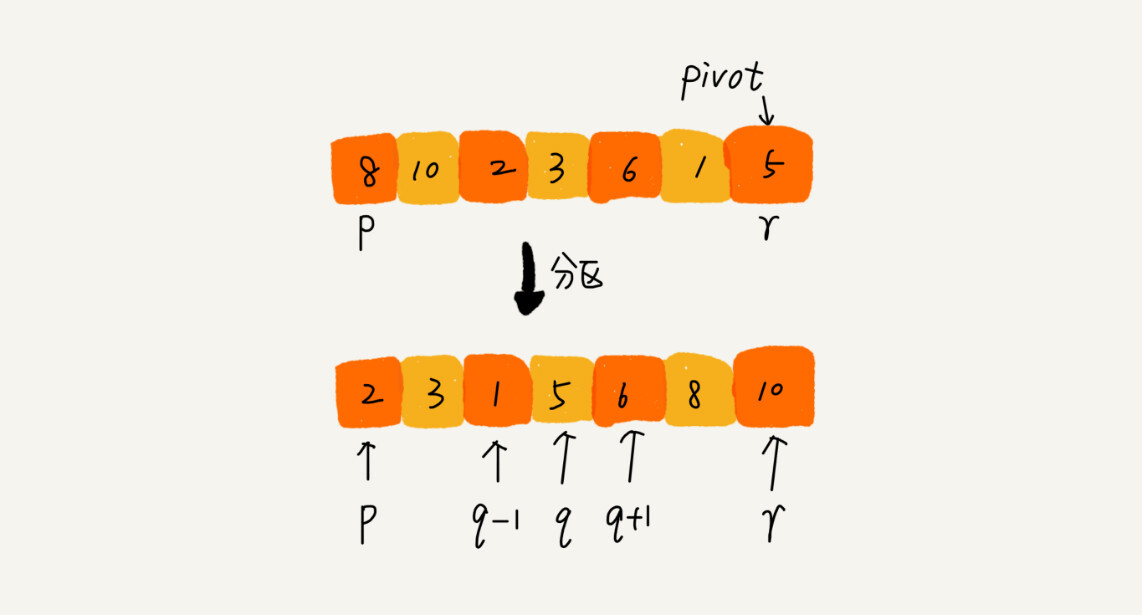``````递推公式：
quick_sort(p…r) = quick_sort(p…q-1) + quick_sort(q+1… r)

p >= r
``````

``````//快速排序 ， a是数组， n表示数组的大小
public void quickSort(int[] a, int n) {
quickSortC(a, 0, n-1);
}
//快速排序递归函数，p，r为下标
public void quickSortC(int[] a, int p, int r) {
if (p >= r) return;

int q = partition(a, p, r); //获取分区点
quickSortC(a, p, q-1);
quickSortC(a, q+1, r);
}
``````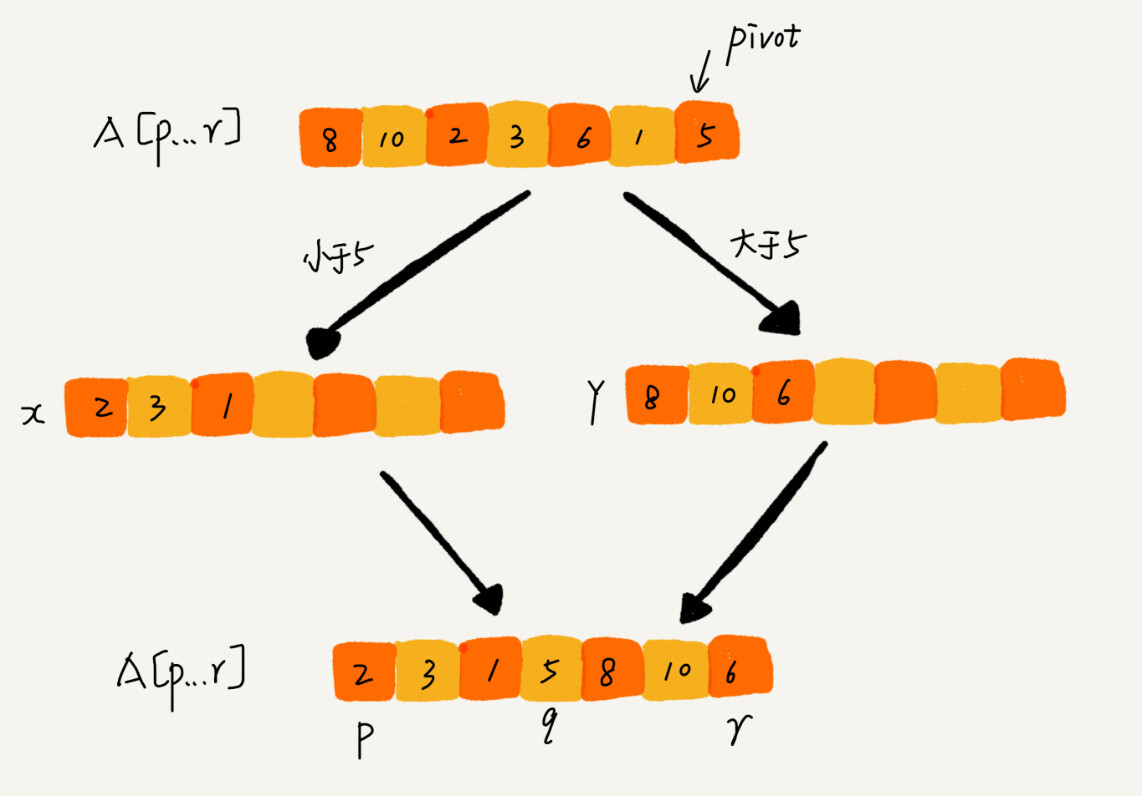``````public int partition(int[] a, int p, int r) {
int pivot = a[r];
int i = p;
int temp = 0;
for (int j = p; j <= r-1; j++) {
if (a[j] < pivot) {
temp = a[i];
a[i] = a[j];
a[j] = temp;
i++;
}
}
temp = a[i];
a[i] = a[r];
a[r] = temp;
return i;
}
``````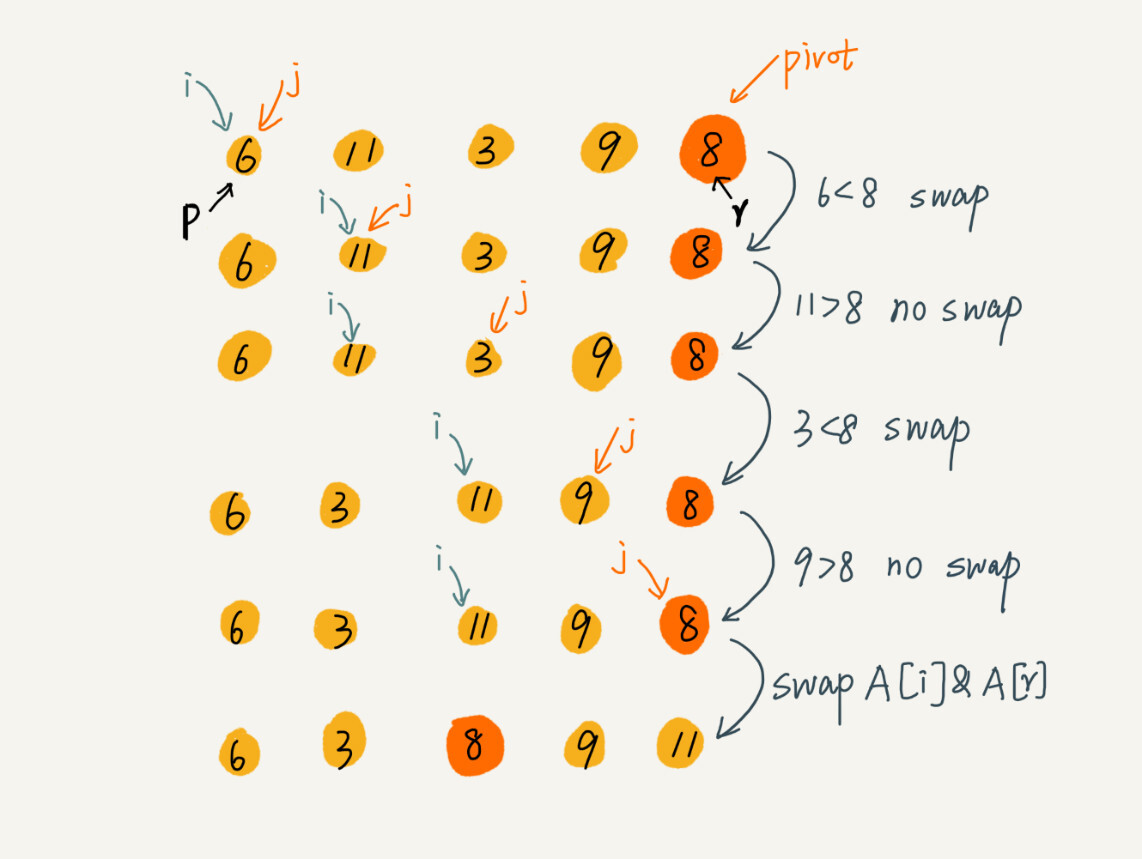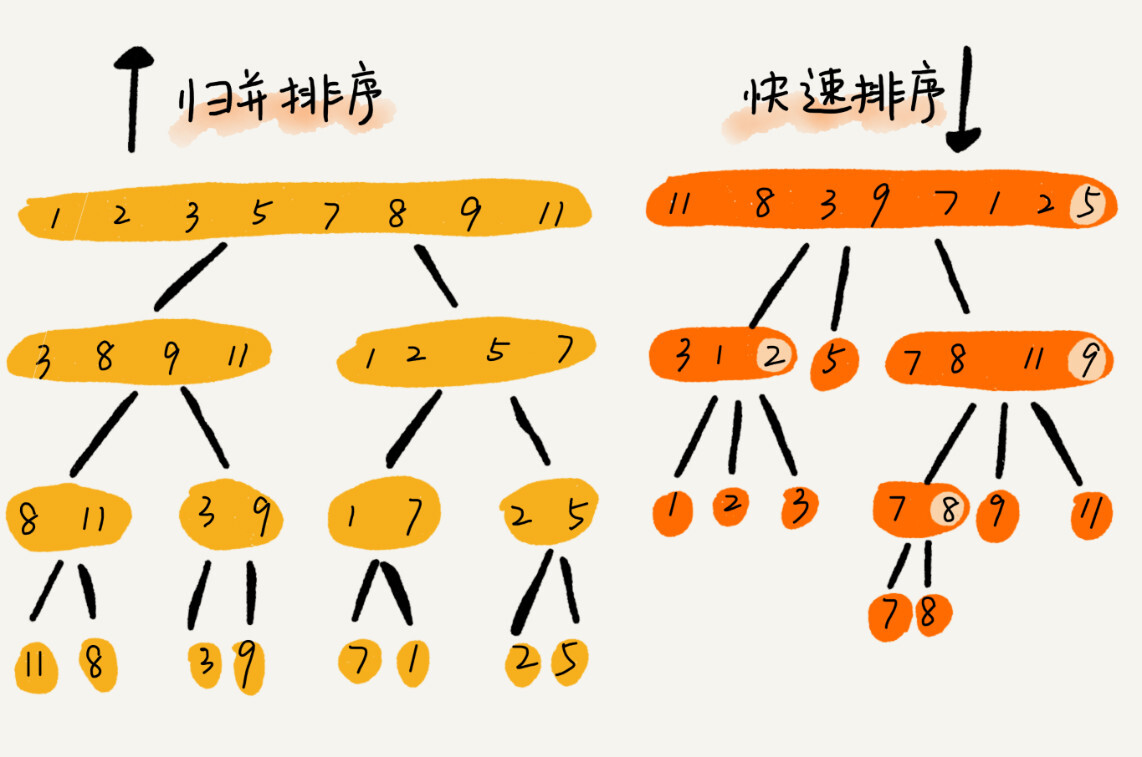#### 快速排序的性能分析

``````T(1) = C；   n=1 时，只需要常量级的执行时间，所以表示为 C。
T(n) = 2*T(n/2) + n； n>1
``````

``````T(1) = C；   n=1 时，只需要常量级的执行时间，所以表示为 C。
T(n) = T(n/10) + T(9*n/10) + n； n>1
``````

#### 解答开篇``````public static int findKthLargest(int[] nums, int k) {
return quickSort(nums, 0, nums.length-1, k);
}

public static int quickSort(int[] nums, int s, int e, int k) {
int pivot = nums[e];
int i = s;
int temp = 0;

for (int j = s; j <= e - 1; j++) {
if (nums[j] > pivot) {
temp = nums[j];
nums[j] = nums[i];
nums[i] = temp;
i++;
}
}
temp = nums[e];
nums[e] = nums[i];
nums[i] = temp;

if (i + 1 == k) {
return nums[i];
} else if (i + 1 < k) {
return quickSort(nums, i+1, e, k);
} else {
return quickSort(nums, s, i-1, k);
}
}
``````

#### 思考

1. 每次从各个文件中取一条数据，在内存中根据数据时间戳构建一个最小堆，然后每次把最小值的数据写入新文件，同时将最小值来自的那个文件再取出来一条数据，加入到最小堆中。这个空间复杂度为O(1)，时间复杂度为O(n)。由于磁盘读取比较耗时，所以可以考虑每次读取一批数据放入内存，没了再从磁盘中读取，时间复杂度还是O(n)。